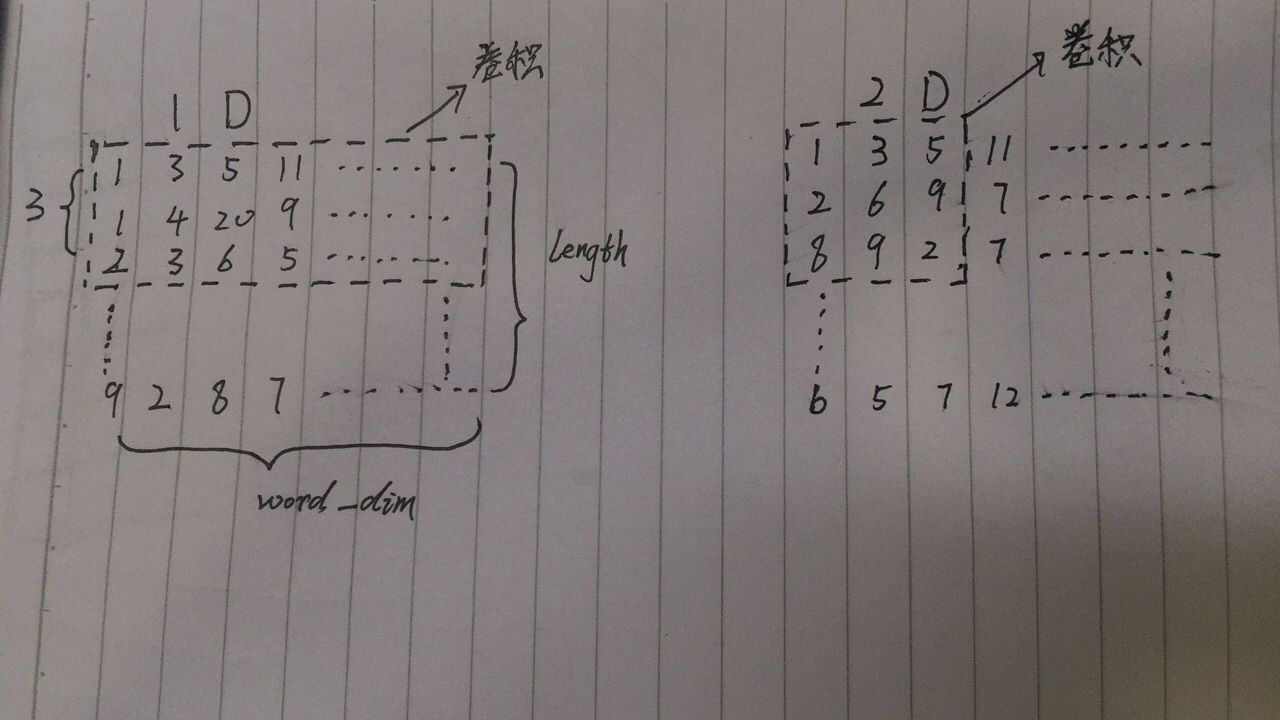# Keras Convolution1D与Convolution2D区别

from keras.layers import Convolution1D
from keras.models import Sequential

model=Sequential()
filters=64,     #64个滤波器 －》生成 64深度
kernel_size=3,  #长度 为 3
input_shape=(10,32),  # 输入形状 就是 图片形状   (step,input_dim) input_dim 一般指词_dim
))
#Output Shape:(None, 10, 64)

filters=32,
kernel_size=3,
))
#Output Shape:(None, 10, 32)  

from keras.layers import Convolution2D
from keras.models import Sequential

model=Sequential()
filters=64, # 64个滤波器 －》生成 64深度
kernel_size=3, # 滤波器窗口 大小（3，3）
input_shape=(256,256,3),  # 输入形状 就是 图片形状  # 默认 data_format:channels_last  (rows,cols,channels)
))
#Output Shape:(None, 256, 256, 64)

filters=32,
kernel_size=3,
))
#Output Shape:(None, 256, 256, 32)

1.Convolution1D主要用于nlp，Convolution2D主要用于cv。实际上，Convolution1D也可以用于cv，Convolution2D也可以用于nlp，只是那个时候不是标准的卷积方式，而是经过一定变形的卷积。

2.可以看到Convolution1D的 只有3这一个参数，Convolution2D 却有两个参数3（即长度为3，宽度为3的卷积窗口）。表面上Convolution1D没有给出卷积的大小，Convolution2D给出了。实际上，在Convolution1D中,长度 为 句子 所有 word 的长度，宽度就是 句子中word的 dim (全称:dimension) ，宽度自动把整个tensor的宽度包裹住。

3.Convolution1D的input_shape是 ( 长度为10，宽度为32 )的tensor。Convolution2D的input_shape是 ( 宽度为256长度为2563个channel ) 的tensor。